# GRE Subject Test: Chemistry : Analytical Chemistry

## Example Questions

1 2 3 4 5 6 7 8 10 Next →

### Example Question #3 : Organic Analyses And Lab Techniques

Extraction is the process of removing compounds from a solution by taking advantage of varying solubilities. The process of extraction for a mixture of diethylamine, phenol, acetic acid, and ammonia involves three steps.

1. Add a strong acid and shake the solution. Let the aqueous layer drain off.

2. Add a weak base and shake the solution. Let the aqueous layer drain off.

3. Add a strong base and shake the solution. Let the aqueous layer drain off.

Which of the following compounds would be the last to enter the aqueous phase using this technique?

Phenol

Diethylamine

Acetic acid

Water

Ammonia

Phenol

Explanation:

Think about what will be reacting with each substance as it is introduced to the solution.

First, a strong acid is added. This will make all bases in the solution (ammonia and diethylamine) become protonated, allowing them to become soluble. They will transition to the aqueous phase and be removed.

Next, a weak base is added. Only carboxylic acids will be deprotonated by this addition, gaining a charge and transitioning to the aqueous phase. Acetic acid will be extracted.

Finally, a strong base will deprotonate the weak acids, such as phenol. As a result, phenol will be the last molecule to leave the solution.

### Example Question #11 : Organic Analyses And Lab Techniques

Which of the following mixtures would not be adequately separated via distillation?

A solution of alcohols of different sizes

Racemic mixture

A solution of polar and nonpolar compounds

A solution containing three diastereomers

Racemic mixture

Explanation:

Distillation involves the separation of compounds by taking advantage of their different boiling points. Mixtures with similar boiling points would not be adequately separated using distillation.

A racemic mixture is a mixture of enantiomers, which have identical boiling points. As a result, distillation would not be effective for separating a racemic mixture.

Diastereomers will have differing physical properties and can be separated by boiling point. Larger alkane chains result in higher boiling points, allowing separation of different sized alcohols by distillation. Polar compounds will have greater intermolecular forces, generally leading to higher boiling points, and allowing them to be separated from nonpolar compounds.

### Example Question #91 : Analytical Chemistry

Which formula gives the relationship between the energy (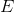) of a photon as a function of the frequency (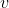) of light?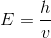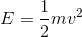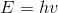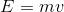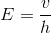Explanation:

There is a direct relationship between energy () of a photon and its frequency (). As energy increases the frequency associated with that photon increases based on the equation(=Planck's constant).

### Example Question #2 : Other Spectroscopy

There are four laser pointers that give off different colors off light. The wavelengths corresponding to these colors are:

violet-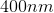red-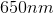green-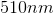blue-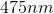yellow-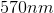Which laser pointer is expected to give off light with the highest energy?

Violet

Blue

Yellow

Green

Red

Violet

Explanation:

Violet waves have the most energy of the visible spectrum because of the relationship: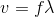Where=speed of light=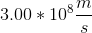,=frequency, and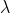=wavelength of light. The equation can be rearranged to:Therefore, there is an inverse relationship between wavelength and frequency. There is a direct relationship between energy and frequency governed by the equation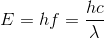Where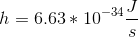is Planck's constant. So as frequency increases, so does the energy of the light waves. Therefore, waves with shorter wavelengths have higher frequencies, and more energy associated with them.

For the violet light: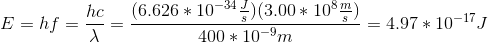If we complete this calculation for the other colors, our conceptual answer will be confirmed mathematically.

### Example Question #3 : Other Spectroscopy

Which analytical method is used primarily to determine the functional groups present in a compound?

Ultraviolet-visible spectroscopy

Mass spectrometry

More than one of these

Infrared (IR) spectroscopy

Nuclear magnetic resonance (NMR) spectroscopy

Infrared (IR) spectroscopy

Explanation:

Infrared (IR) spectroscopy is used to determine the functional groups present in a molecule. It makes use of the IR region of the electromagnetic spectrum. When an analyte is exposed to IR radiation, different functional groups absorb radiation at their characteristic wavelengths causing vibrational and rotational excitation to bonds within the molecule. The frequencies absorbed correspond to the amount of energy required to increase the molecular vibration of the functional groups in a molecule and can be plotted on a graph for analysis. Mass spectroscopy is used primarily to determine the amount and types of chemicals present within a sample. Ultraviolet-visible spectroscopy is used to analyze highly conjugated compounds, biological molecules, and transition metals. NMR spectroscopy gives information about the electronic structure of a molecule.

### Example Question #92 : Analytical Chemistry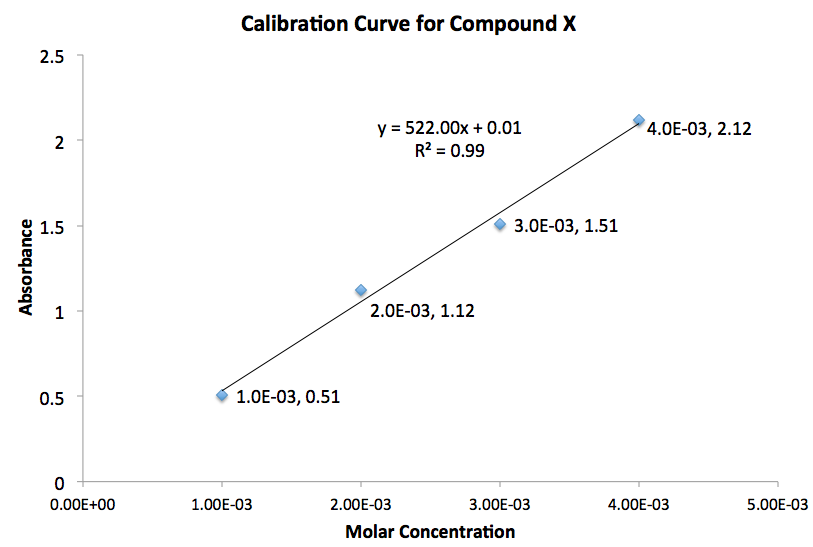In a UV-visible absorption spectroscopy experiment, the length of the cell or cuvette is 1cm. Given the calibration curve for a substance, what is the molar extinction coefficient for this compound?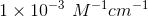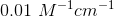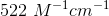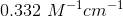Explanation:

The equation for Beer's law is: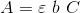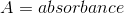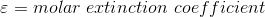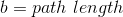Rearrange the equation: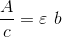Given that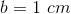, the slope of the line in the graph provided equals to the molar extinction coefficient. Therefore, the answer is1 2 3 4 5 6 7 8 10 Next →

### All GRE Subject Test: Chemistry Resources